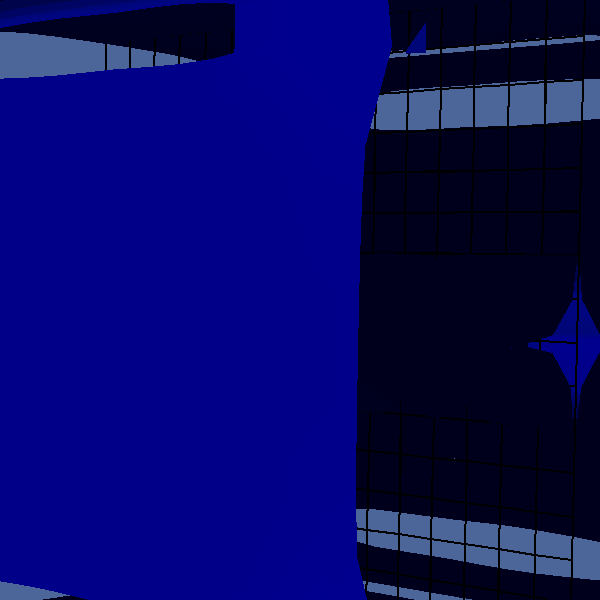# The fractal dimension of a coil

Similar to the analysis that was done for the Koch fractal curve, the fractal dimension of a coil is studied.

#include "grid/octree.h"
#include "utils.h"

int main(){

First we calculate 150000 points on a coil. The ratio between the radius and winding distance is approx. R/d=40, there are about 60 windings in total.

  int jmax=150000;
double jm,jmd;
double xn[jmax],yn[jmax],zn[jmax];
jm= (double) jmax;
for (int j=0;j<jmax;j++){
jmd = (double) j;
xn[j]=0.75*sin((jmd/jm)*3.14*120.);
yn[j]=0.75*cos((jmd/jm)*3.14*120.);
zn[j]=2.*(((jmd)/jm)-0.5);
}
FILE * fp1 = fopen("cyl.dat","w");
for (int j=0;j<jmax;j++){
fprintf(fp1,"%g %g %g\n",xn[j],yn[j],zn[j]);
}
fclose(fp1);
The plot below shows the parameterized coil.
  set xr [-1.2:1.2]
set yr [-1.2:1.2]
set view equal xyz
set xlabel 'z'
set ylabel 'x'
set zlabel 'y'
splot 'cyl.dat' using 3:1:2 title 'Coil' with lines(script)

Looks OK, now the algorithm is applied.

  FILE * fpit = fopen("cells.dat","w");
L0=2.5;
X0=-L0/2;
Y0=X0;
Z0=X0;
init_grid(1<<3);
scalar c[];
for (int m=0;m<7;m++){
foreach(){
c[]=0.;
}
for(int i=0;i<jmax;i++){
Point point = locate(xn[i],yn[i],zn[i]);
if (point.level>0)
c[]+=1.0;
}
int gcc=0;
int gcn=0;
foreach(reduction(+:gcc) reduction(+:gcn)){
gcn++;
if(c[]>0.5)
gcc++;
}
fprintf(fpit,"%d\t%d\t%d\n",m,gcc,gcn);
fflush(fpit);

boundary({c});
boundary({c});
int i=m;
scalar lev[];
foreach()
lev[]=level;
static FILE * fp3 =
popen ("gfsview-batch3D coil.hop.gfv | ppm2gif > fracz.gif --delay 100","w");
output_gfs(fp3);
fprintf (fp3, "Save stdout { format = PPM width = 600 height = 600}\n");
fflush(fp3);
fprintf(ferr,"%d\t",m);
}
}

## Results

First a visualization of the c=1 isosurface is given:Visualization of the refinement iterations with the c=1 isosurface, colored with the level of refinement. The results form the last iteration are poorly displayed as it entails data at a sub-pixel resolution

It appears that the isosurface starts as a cylinder before the coil structre is retrieved at a higher resolution.

Now we study the number of grid cells that lay on the curve as a function of the refinement iteration.

set xr [ -0.5:6.5]
set yr [100:100000]
set logscale y
set xlabel 'refinement iteration'
set ylabel 'Grid cells on the curve'
set key top left box 3
plot "cells.dat" title "Number of cells on the curve" ,\
(2**(1*x))*1200 lw 2 title "1D scaling",\
(2**(2*x))*150 lw 2 title "2D scaling"(script)

For the coarser resolutions the coil appears to be two-dimensional, wheareas at higher resolutions the curve seems to be one-dimensional. This corresponds with the transition from a cylinder-like (i.e. 2D) appearance of the coil at coarser resolutions to the line (i.e. 1D) apearance a finer resolutoins.